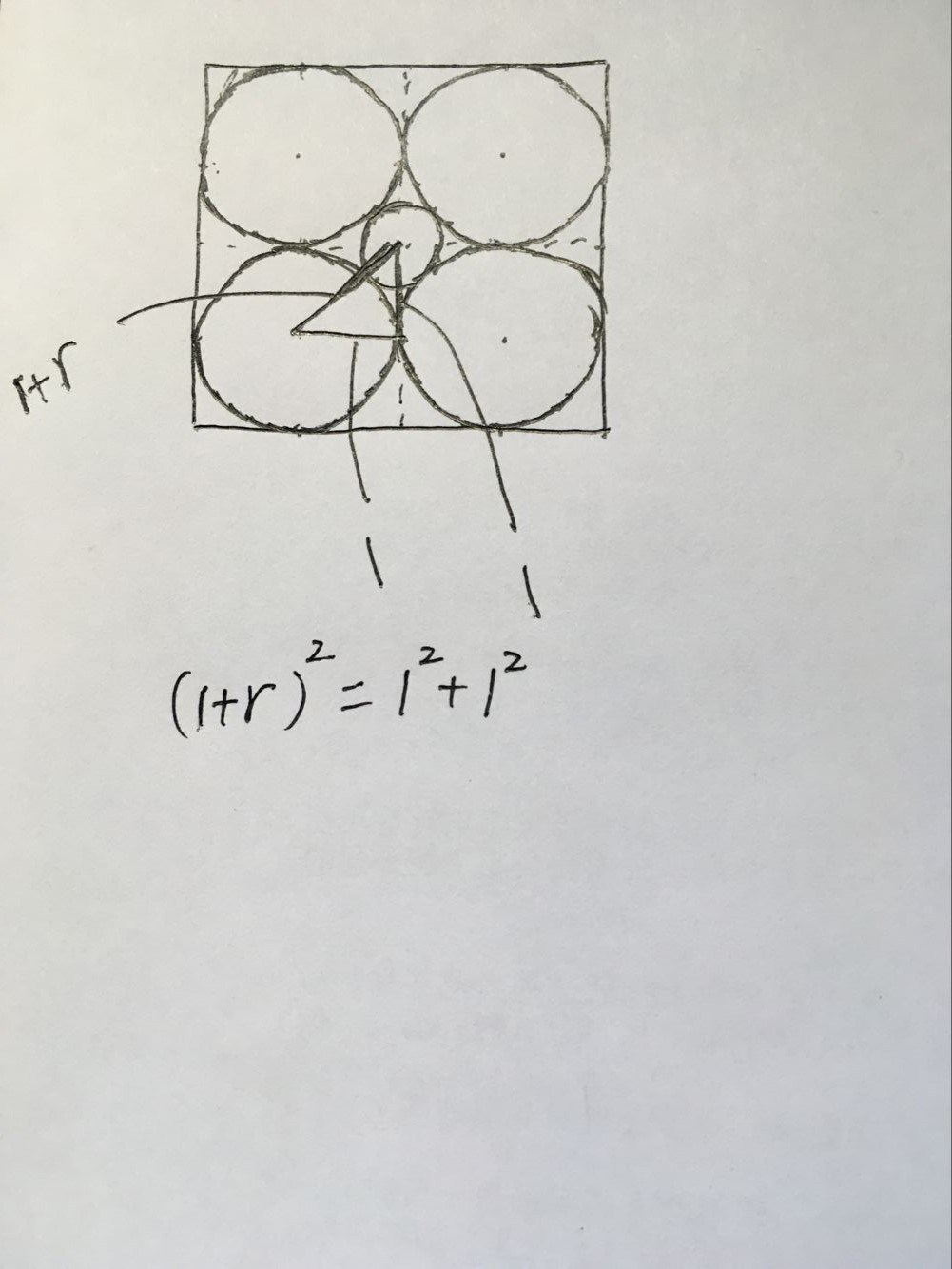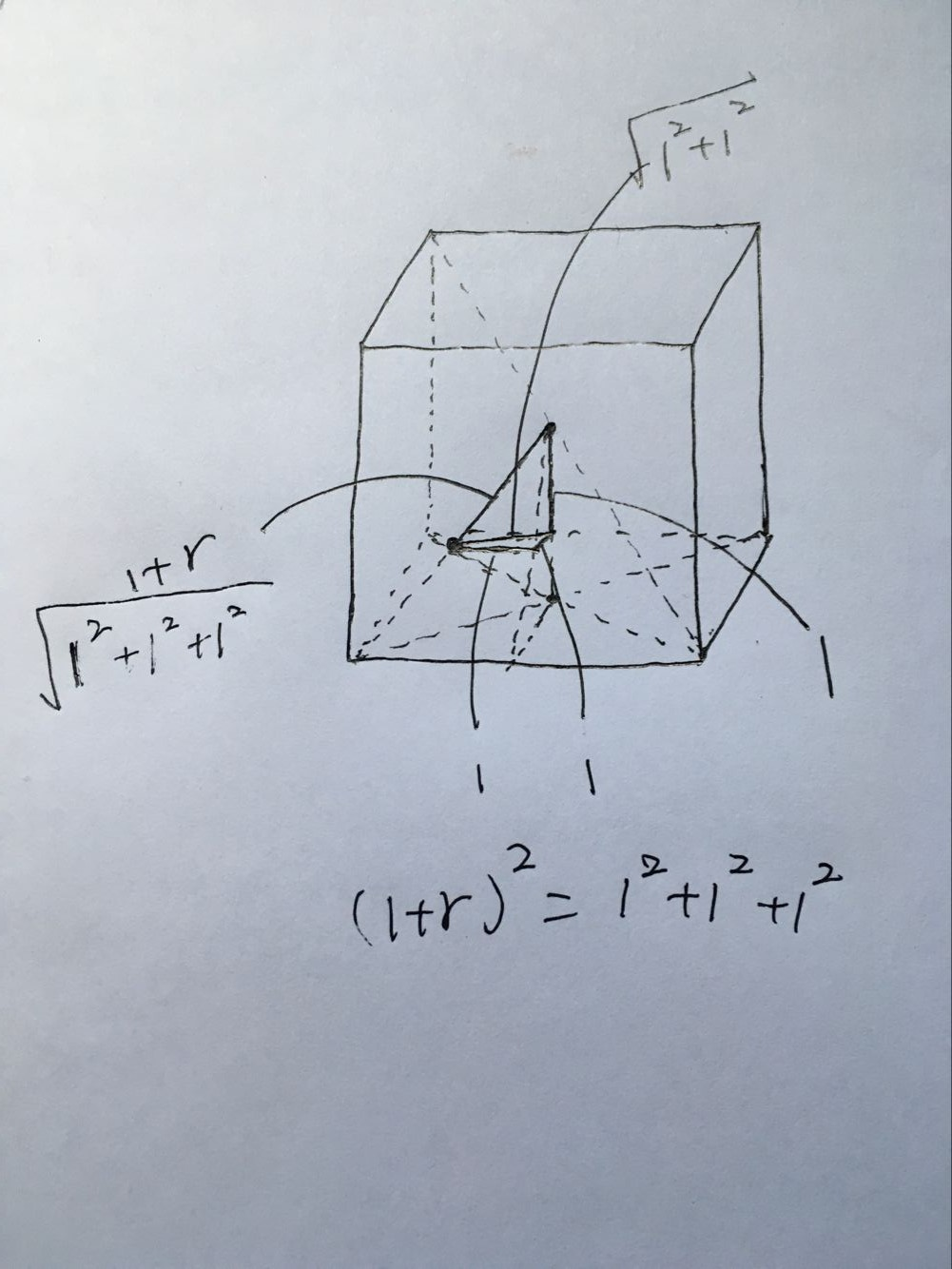# 从几何角度切入最近邻

K近邻

d(a,b)=[ d=1 D (a d b d ) 2 ] 12

KNN-PREDICT(D,K,xHat)
S <- []
for n = 1 to N do
s <- s + <d(xn, xHat), n>       //存储与第n个样本的距离
end for
S <- SORT(S)                        //排序，将最近的放在前面
yHat <- 0
for k = 1 to K do
<dist, n> <- Sk                 //n是第k个最近的数据点
yHat <- yHat + yn               //用第n个数据点的类别进行投票
end for
return SIGN(yHat)                   //如果yHat > 0 返回1，否则返回-1


K均值聚类

K均值聚类算法非常简单，并且能有效进行聚类。它的主要思想是，将每一个类（簇）用它的簇心来表示，有了这个簇心，我们就很容易将一个数据点分配给最近的那个簇。相似地，如果我们知道数据点属于哪个簇，我们很容易计算出簇心。这有点像是先有鸡还是先有蛋的问题，如果我们知道是哪个簇，我们可以计算簇心，而如果我们知道簇心，就能计算属于哪个簇。但是这两个我们都不知道。

K-Means
for k = 1 to K do
uk <- some random location              //随机初始化k个簇心
end for
repeat
for n = 1 to N do
zn <- argmink||uk - xn||            //计算样本n最近的簇心
end for
for k = 1 to K do
Xk <- {xn: zn = k}                  //属于簇k的数据点
uk <- MEAN(Xk)                      //重新计算簇心
end for
util us stop changing                       //直到簇心不再发生变化
return z                                    //返回属于哪个簇avgDist(D)= N i=1  N j=1 ||d i d j ||N 2